Courses

# NCERT Solutions(Part - 3) - Fractions and Decimals Class 7 Notes | EduRev

## Mathematics (Maths) Class 7

Created by: Praveen Kumar

## Class 7 : NCERT Solutions(Part - 3) - Fractions and Decimals Class 7 Notes | EduRev

The document NCERT Solutions(Part - 3) - Fractions and Decimals Class 7 Notes | EduRev is a part of the Class 7 Course Mathematics (Maths) Class 7.
All you need of Class 7 at this link: Class 7

Exercise 2.6
Ques 1:
Find:
(i) 0.2 x 6
(ii) 8 x 4.6
(iii) 2.71 x 5
(iv) 20.1 x 4
(v) 0.05 x 7
(vi) 211.02 x 4
(vii) 2 x 0.86
Ans:
(i) 0.2 x 6 = 1.2
(ii) 8 x 4.6 = 36.8
(iii) 2.71 x 5 = 13.55
(iv) 20.1 x 4 = 80.4
(v) 0.05 x 7 = 0.35
(vi) 211.02 x 4 = 844.08
(vii) 2 x 0.86 = 1.72

Ques 2: Find the area of rectangle whose length is 5.7 cm and breadth is 3 cm.
Ans: Given: Length of rectangle = 5.7 cm and
Breadth of rectangle = 3 cm
Area of rectangle = Length x Breadth = 5.7 x 3 = 17.1 cm2
Thus, the area of rectangle is 17.1 cm2.

Ques 3:
Find:
(i) 1.3 x 10
(ii) 36.8 x 10
(iii) 153.7 x 10
(iv) 168.07 x 10
(v) 31.1 x 100
(vi) 156.1 x 100
(vii) 3.62 x 100
(viii) 43.07 x 100
(ix) 0.5 x 10
(x) 0.08 x 10
(xi) 0.9 x 100
(xii) 0.03 x 1000
Ans:
(i) 1.3 x 10 = 13.0
(ii) 36.8 x 10 = 368.0
(iii) 153.7 x 10 = 1537.0
(iv) 168.07 x 10 = 1680.7
(v) 31.1 x 100 = 3110.0
(vi) 156.1 x 100 = 15610.0
(vii) 3.62 x 100 = 362.0
(viii) 43.07 x 100 = 4307.0
(ix) 0.5 x 10 = 5.0
(x) 0.08 x 10 = 0.80
(xi) 0.9 x 100 = 90.0
(xii) 0.03 x 1000 = 30.0

Ques 4: A two-wheeler covers a distance of 55.3 km in one litre of petrol. How much distance will it cover in 10 litres of petrol?
Ans:
∵ In one litre, a two-wheeler covers a distance = 55.3 km
∴ In 10 litres, a two- wheeler covers a distance = 55.3x10 = 553.0 km
Thus, 553 km distance will be covered by it in 10 litres of petrol.

Ques 5:
Find:
(i) 2.5 x 0.3
(ii) 0.1 x 51.7
(iii) 0.2 x 316.8
(iv) 1.3 x 3.1
(v) 0.5 x 0.05
(vi) 11.2 x 0.15
(vii) 1.07 x 0.02
(viii) 10.05 x 1.05
(ix) 101.01 x 0.01
(x) 100.01 x 1.1
Ans:
(i) 2.5 x 0.3 = 0.75
(ii)  0.1 x 51.7 = 5.17
(iii) 0.2 x 316.8 = 63.36
(iv) 1.3 x 3.1=4.03
(v) 0.5 x 0.05 =0.025
(vi) 11.2 x 0.15 = 1.680
(vii) 1.07 x 0.02 = 0.0214
(viii) 10.05 x 1.05 = 10.5525
(ix) 101.01 x 0.01 = 1.0101
(x) 100.01 x1.1=110.11

Exercise 2.7
Ques 1:
Find:
(i) 0.4 ÷ 2
(ii) 0.35 ÷ 5
(iii) 2.48 ÷ 4
(iv) 65.4 ÷ 6
(v)  651.2 ÷ 4
(v)  14.49 ÷ 7
(vii) 3.96 ÷ 4
(viii) 0.80 ÷ 5
Ans:
(i)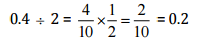(ii)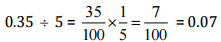(iii)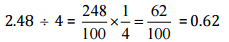(iv)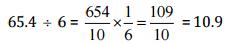(v)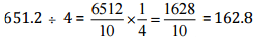(vi)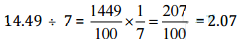(vii)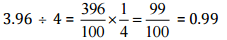(viii)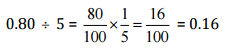Ques 2:
Find:
(i) 4.8 ÷ 10
(ii) 52.5 ÷ 10
(iii) 0.7 ÷ 10
(iv) 33.1 ÷ 10
(v) 272.23 ÷ 10
(vi) 0.56 ÷ 10
(vii) 3.97 ÷ 10
Ans:
(i)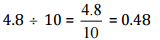(ii)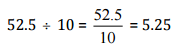(iii)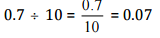(iv)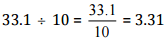(v)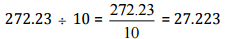(vi)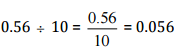(vii)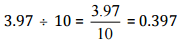Ques 3:
Find:
(i) 2.7 ÷ 100
(ii)  0.3 ÷ 100
(iii) 0.78 ÷ 100
(iv) 432.6 ÷ 100
(v) 23.6 ÷ 100
(vi) 98.53 ÷ 100
Ans:
(i)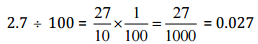(ii)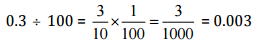(iii)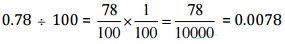(iv)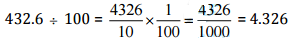(v)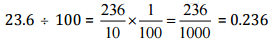(vi)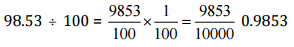Ques 4:
Find:
(i) 7.9 ÷ 1000
(ii)  26.3 ÷ 1000
(iii) 38.53 ÷ 1000
(iv) 128.9 ÷ 1000
(v)  0.5 ÷ 1000
Ans:
(i)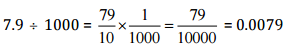(ii)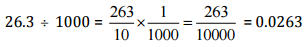(iii)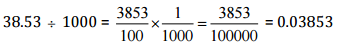(iv)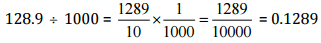(v)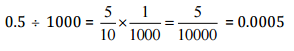Ques 5:
Find:
(i) 7÷ 3.5
(ii)  36 ÷ 0.2
(iii)  3.25  ÷  0.5
(iv)  30.94 ÷ 0.7
(v)  0.5 ÷ 0.25
(vi)  7.75  ÷  0.25
(vii) 76.5 ÷ 0.15
(viii)  37.8 ÷1.4
(ix)  2.73  ÷  1.3
Ans:
(i)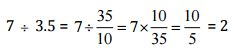(ii)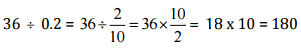(iii)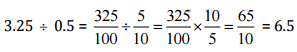(iv)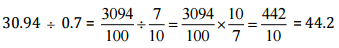(v)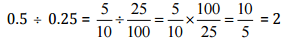(vi)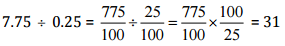(vii)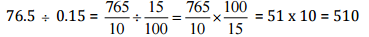(viii)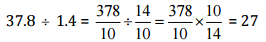(ix)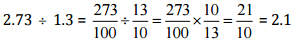Ques 6: A vehicle covers a distance of 43.2 km in 2.4 litres of petrol. How much distance will it cover in one litre petrol?
Ans:
∵ In 2.4 litres of petrol, distance covered by the vehicle = 43.2 km
∴ In 1 litre of petrol, distance covered by the vehicle = 43.2 ÷2.4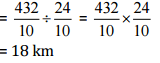Thus, it covered 18 km distance in one litre of petrol.

Offer running on EduRev: Apply code STAYHOME200 to get INR 200 off on our premium plan EduRev Infinity!

,

,

,

,

,

,

,

,

,

,

,

,

,

,

,

,

,

,

,

,

,

;